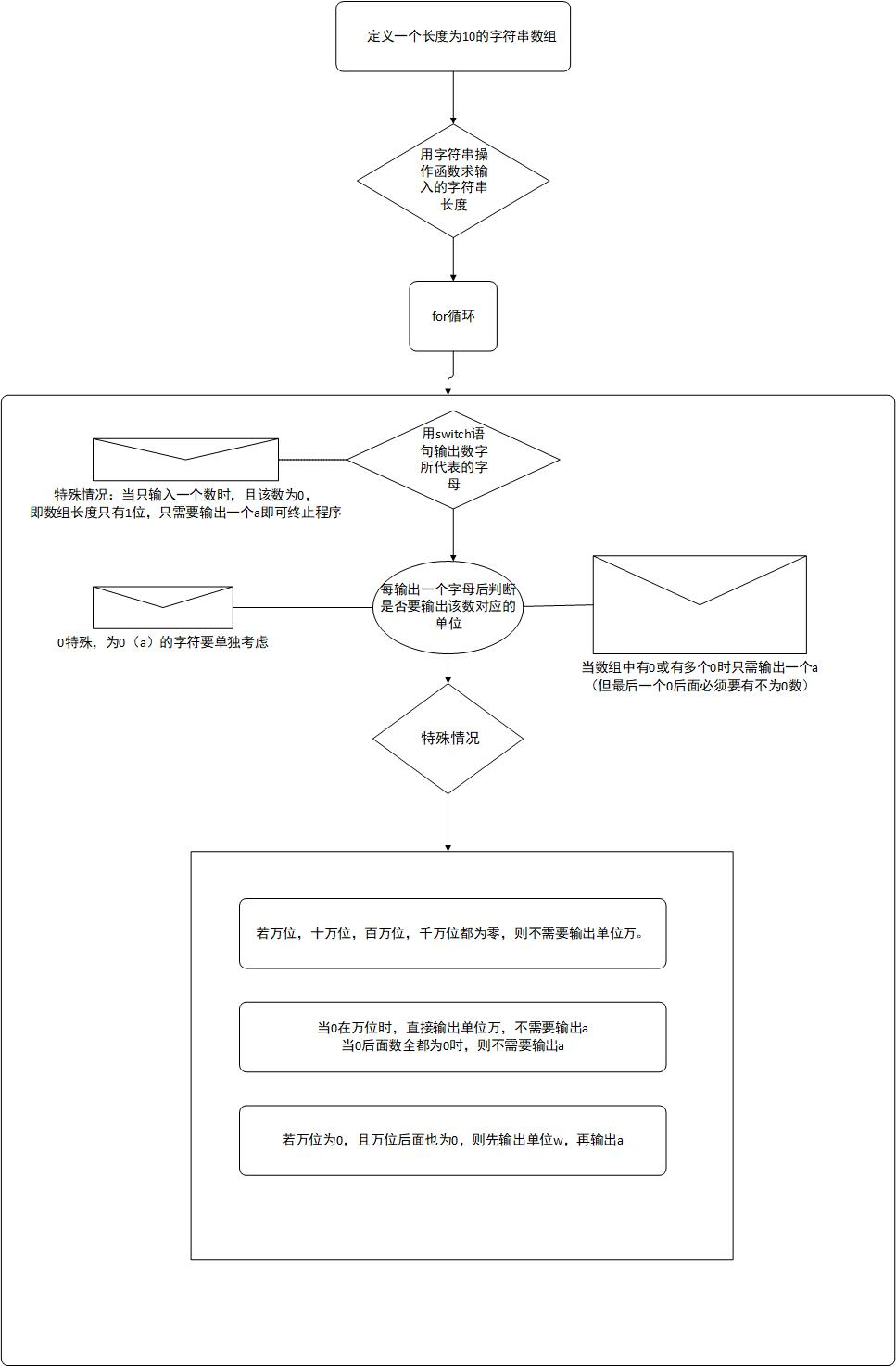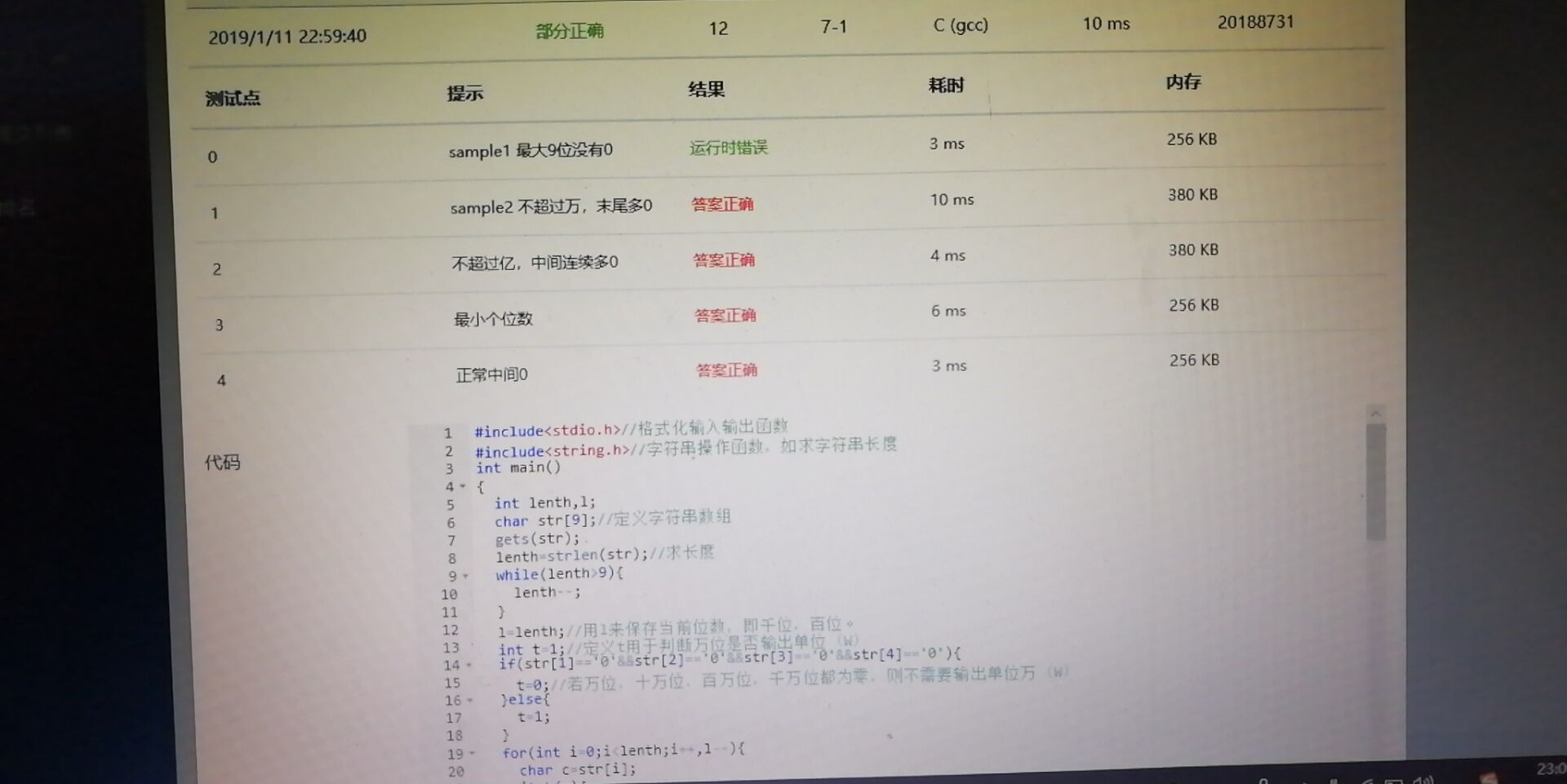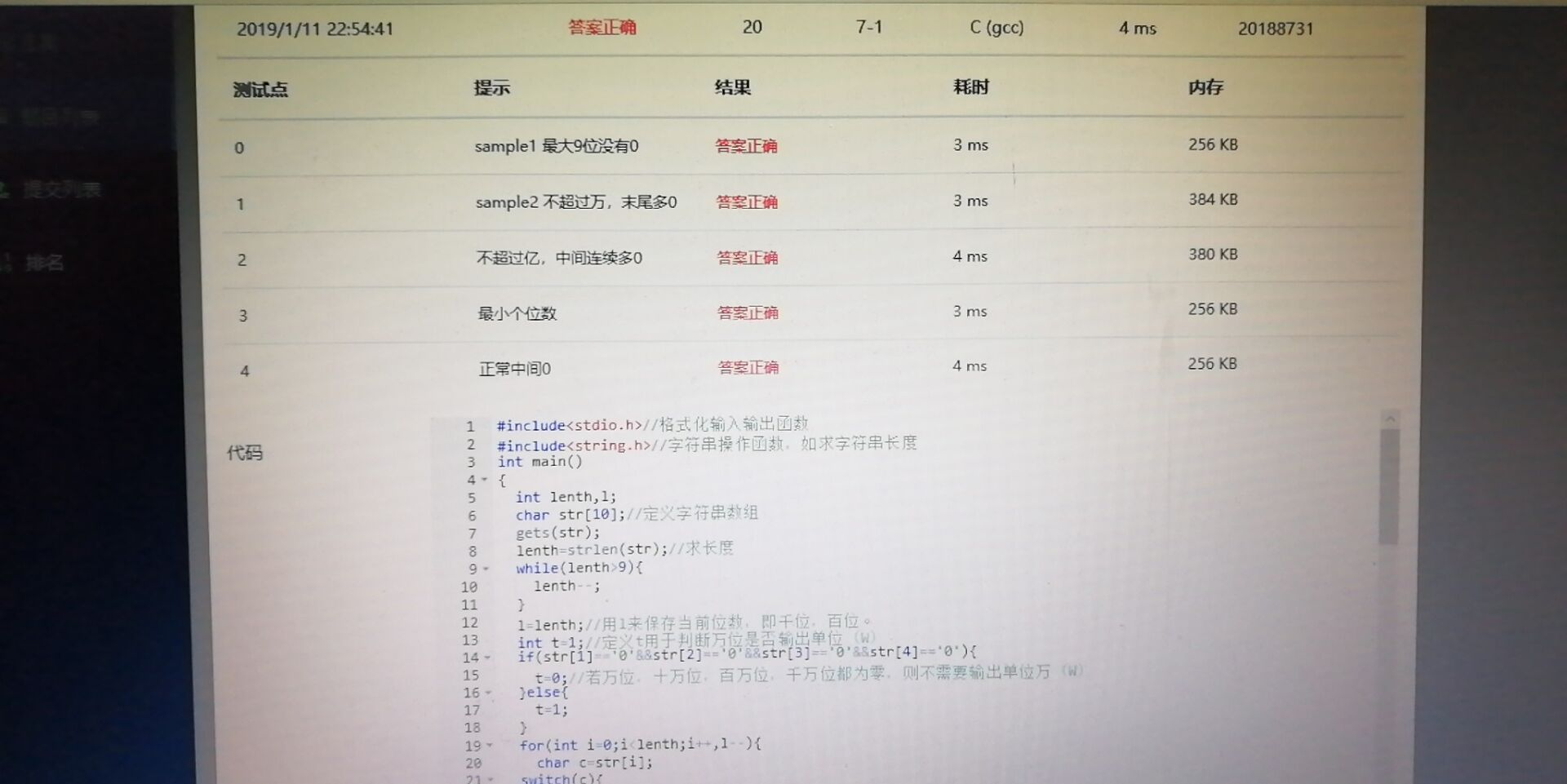7-1 币值转换 （20 分）

813227345

iYbQdBcScWhQdBeSf

6900

gQjB

(一)实验代码：

#include<stdio.h>//格式化输入输出函数
#include<string.h>
int main()
{
int l,n;
char str;//定义字符串数组
gets(str);
l=strlen(str);//求长度
while(l>9){
l--;
}
n=l;
int t=0;//定义t用于判断万位是否输出单位（W）
if(str=='0'&&str=='0'&&str=='0'&&str=='0'){
t=1;//若万位，十万位，百万位，千万位都为零，则不需要输出单位万（W）
}else{
t=0;
}
for(int i=0;i<l;i++,n--){
char c=str[i];
switch(c){
case'0':c='a';break;
case'1':c='b';break;
case'2':c='c';break;
case'3':c='d';break;
case'4':c='e';break;
case'5':c='f';break;
case'6':c='g';break;
case'7':c='h';break;
case'8':c='i';break;
case'9':c='j';break;
default:break;
}
if(l==1&&str=='0'){
printf("a");
return 0;
}
if(c=='a'){//0特殊，为0（a）的字符单独考虑
if(l==5&&t){
printf("W");//当0在万位时，直接输出单位万，不需要输出零（a）
}else{//当0不在万位时
for(i++,l--;i<l;i++,l--){//看0的下几位是否为0
if(str[i]=='0'){
if(n==5&&t){
printf("W");
}
}
else{
printf("a");
l++;i--;/*将l和i的值恢复到上一位为0的数所代表的值，因为break后，上面的for循环又要把l和i的
恢复到先前那个不为0的值所代表的值 */
break;
}
}
}
}else{//字符不为0（a）。
printf("%c",c);//输出字符
switch(n){//位数
case 9:printf("Y");break;
case 8:case 4:printf("Q");break;
case 7:case 3:printf("B");break;
case 6:case 2:printf("S");break;
case 5:printf("W");break;
default:break;
}
}
}
return 0;
}

2、设计思路
----------3、实验中遇到的问题及解决方案4、运行结果截图
----------posted @ 2019-02-14 08:56 小嗝嗝爱作业 阅读(...) 评论(...) 编辑 收藏